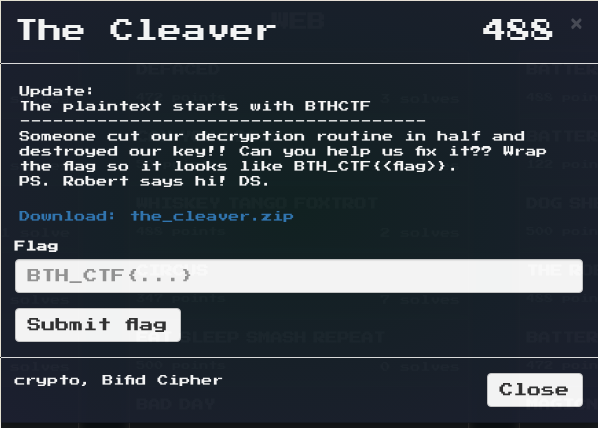# The Cleaver

## Challenge Description

The challenge supplied source code to a bifid cipher and a ciphertext.txt### Ciphertext

  BYOITAAVOBSOSODVZTEOUBFNCBSOTONVGP


### Source code

#!/usr/bin/python
import numpy as np

class BifidCipher():
def __init__(self, key=None):

if key is None:
key = self.genKey()

self.key = key

def genKey(self):
ALPHABET = "ABCDEFGHIKLMNOPQRSTUVWXYZ"
matrix = np.random.choice(range(len(ALPHABET)),(5,5), replace=False)

key = []
for i in range(5):
row = []
for j in range(5):
row.append(ALPHABET[matrix[i][j]])
key.append(row)

return np.array(key)

def encrypt(self, plaintext):

plaintext = plaintext.replace("J", "I")

rows = ""
columns = ""
for char in plaintext:
location = np.where(self.key==char)
rows += str(location)[1:-1]
columns += str(location)[1:-1]

pre_group = rows+columns

ciphertext = ""
for i in xrange(0, len(pre_group), 2):
row = int(pre_group[i])
column = int(pre_group[i+1])
ciphertext+=self.key[row][column]

return ciphertext

# HAHA YOU CAN NEVER FIX THIS
def decrypt(self, ciphertext):
ciphertext_int
for char in ciphertext:
location = np.where(self.k
row = str(location[0
column = str(location[]
ciphertext_int += ro

plaintext=
limit = len(ciphertext_in
for i in range(limit/2
left_side = int(ciphertext_i
right_side = int(ciphertext_int[i+li
plaintext += self.key[le

return plaintext

# Hmmm what to place in the question marks :thinking_emoji:
key = np.array([['W', 'I','Q','?','M'],
['?','F','?','U','Y'],
['X', 'A', 'Z', 'R', 'E'],
['S', 'O', 'G', '?', 'K'],
['?', 'V', 'L', 'P', 'C']])

f = open("ciphertext.txt", "r")
ciphertext = f.read()
f.close()

bc = BifidCipher(ciphertext)
plaintext = bc.decrypt()

print "Flag: ", plaintext



### The attack

When running the challenge we get

  File "./bifidcipher.py", line 51
row = str(location[0
^
SyntaxError: invalid syntax


And when reading the description we see that the decryption routine is literally cut in half. Ok, we fix it. You can either search for Bifid cipher on google and reimplement it, or just fix the code.

### Fixed decryption routine

 # HAHA YOU CAN NEVER FIX THIS. YES I CAN
def decrypt(self, ciphertext):
ciphertext_int = ""
for char in ciphertext:
location = np.where(self.key==char)
row = str(location)[1:-1]
column = str(location)[1:-1]
ciphertext_int += row+column

plaintext=""
limit = len(str(ciphertext_int))
for i in range(limit/2):
left_side = int(ciphertext_int[i])
right_side = int(ciphertext_int[i+limit/2])
plaintext += self.key[left_side][right_side]

return plaintext


So now we just need to fix the key.

We see that there are five letters missing in the key. B, T, H, N and D.

## Solution

Now that we have our missing letters and fixed code, we can easily fix the key. We simply list all permutations of the key and our missing letters and print each one of the possible solutions.

from itertools import permutations
missing = "BTHND"
possible_solutions = list(permutations(missing))

for solution in possible_solutions:
key = np.array([['W', 'I','Q',solution,'M'],
[solution,'F',solution,'U','Y'],
['X', 'A', 'Z', 'R', 'E'],
['S', 'O', 'G', solution, 'K'],
[solution, 'V', 'L', 'P', 'C']])

bc = BifidCipher(key)
plaintext = bc.decrypt(ciphertext)

print plaintext


We print the output to a file and grep for the flag format. BTHCTF

$./bifidcipher.py > solutions.txt$ cat solutions.txt | grep "BTHCTF"


And we get the flag!

BTHCTFIUSTAFANCYSUBSTITUTIONCIPHER